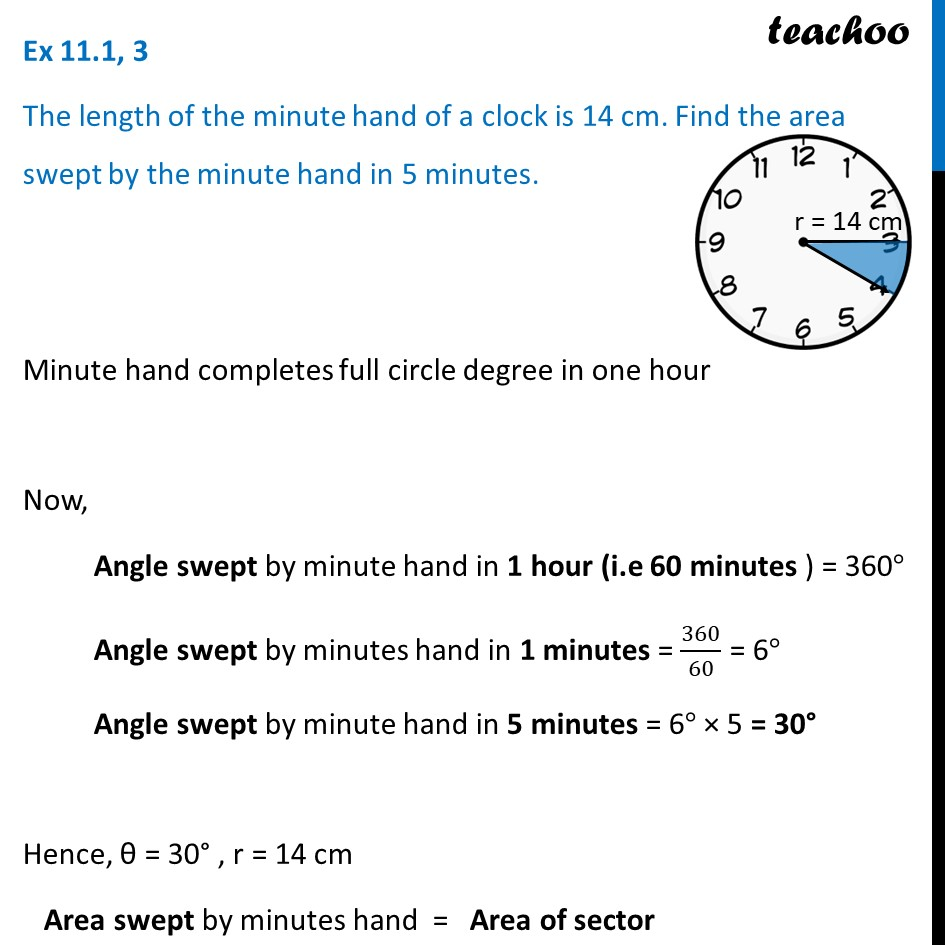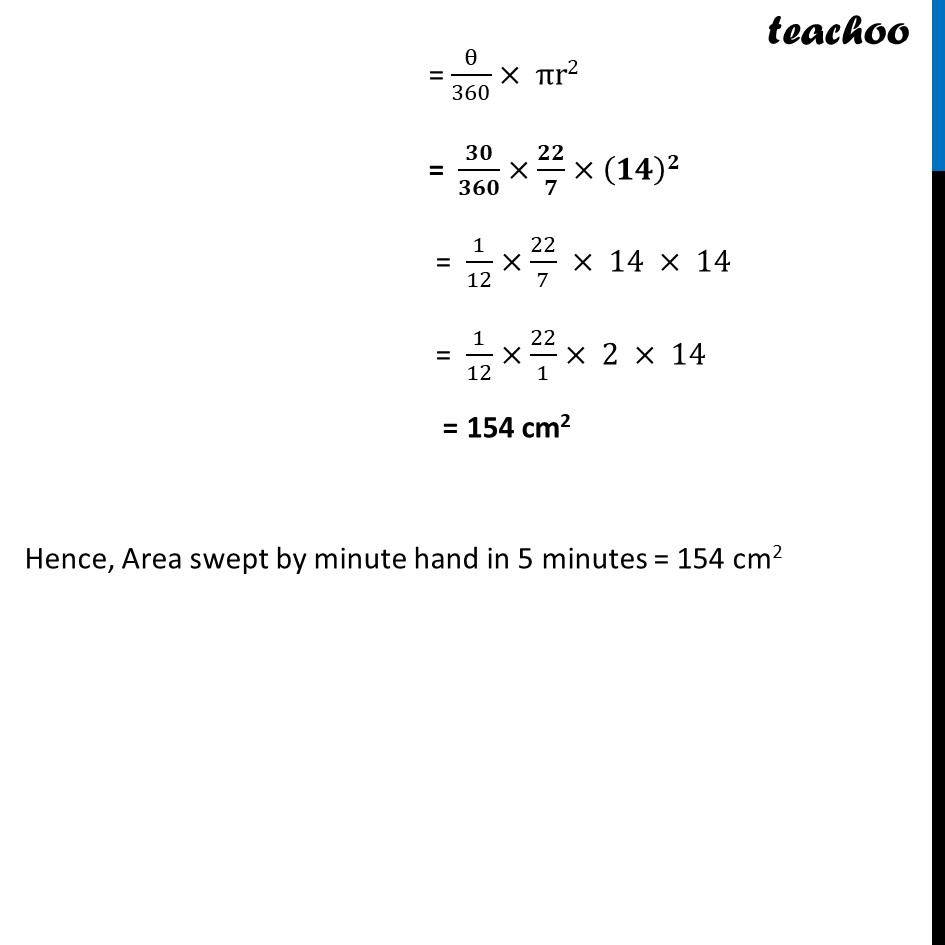Area of sector of circle

Chapter 11 Class 10 Areas related to Circles
Concept wiseLearn in your speed, with individual attention - Teachoo Maths 1-on-1 Class

### Transcript

Ex 11.1, 3 The length of the minute hand of a clock is 14 cm. Find the area swept by the minute hand in 5 minutes. Minute hand completes full circle degree in one hour Now, Angle swept by minute hand in 1 hour (i.e 60 minutes ) = 360° Angle swept by minutes hand in 1 minutes = 360/60 = 6° Angle swept by minute hand in 5 minutes = 6° × 5 = 30° Hence, θ = 30° , r = 14 cm Area swept by minutes hand = Area of sector = θ/360× πr2 = 𝟑𝟎/𝟑𝟔𝟎×𝟐𝟐/𝟕×(𝟏𝟒)𝟐 = 1/12×22/7 × 14 × 14 = 1/12×22/1× 2 × 14 = 154 cm2 Hence, Area swept by minute hand in 5 minutes = 154 cm2